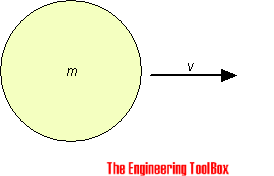Engineering ToolBox - Resources, Tools and Basic Information for Engineering and Design of Technical Applications!

# Conservation of Momentum

## The momentum of a body is the product of its mass and velocity - recoil calculator.

### Linear Momentum

The momentum of a body is defined as the product of its mass and velocity. Since velocity is a vector quantity - momentum is a vector quantity.The momentum of a body can be expressed as

ML = m v                                      (1)

where

ML = linear momentum (kg m/s, lb ft/s)

m = mass of body (kg, lb)

v = velocity of body (m/s, ft/s)

The momentum of a body remains the same as long as there is no external forces acting on it. The principle of conservation of momentum can be stated as

the total linear momentum of a system is a constant

The total momentum of two or more bodies before collision in a given direction is equal to the total momentum of the bodies after collision in the same direction and can be expressed as

ML = m1 v1 + m2 v2 + ..  + mn vn

=  m1 u1 + m2 u2 + ..  + mn un                                       (2)

where

v = velocities of bodies before collision (m/s, ft/s)

u = velocities of bodies after collision (m/s, ft/s)

#### Example - Linear Momentum

A body with mass 30 kg and velocity 30 m/s collides with a body with mass 20 kg and velocity 20 m/s. The velocities of both bodies are in the same direction. Assuming the both bodies have same velocity after impact - the resulting velocity can be calculated as:

ML = (30 kg) (30 m/s)  + (20 kg) (20 m/s)

=  u ((30 kg) + (20 kg))

= constant

transformed:

u = (30 kg) (30 m/s)  + (20 kg) (20 m/s)  /  ((30 kg) + (20 kg))

= 26 (m/s)

#### Example - Recoil Velocity after Rifle Shoot

A rifle weighing 6 lb fires a bullet weighing 0.035 lb with muzzle speed 2100 ft/s. The total momentum of the rifle and bullet can be expressed as

ML = mr vr + mb vb

= mr ur + mb ub

The rifle and the bullet are initially at rest (vr = vb = 0):

ML = (6 lb) (0 ft/s) + (0.035 lb) (0 ft/s)

= (6 lb) ur (ft/s) + (0.035 lb) (2100 ft/s)

= 0

The rifle recoil speed:

ur = - (0.035 lb) (2100 ft/s) / (6 lb)

= - 12.25 (ft/s)

The recoil kinetic energy in the rifle can be calculated as

E = 1/2 m ur2

= 1/2 (6 lb) (-12.25)2

= 450 lb ft

The force required to slow down a recoil depends on the slow down distance. With a slow down distance s = 1.5 in (0.125 ft) - the acting force can be calculated as

F = E / s

= (450 lb ft) / (0.125 ft)

= 3600 lb

#### Recoil Calculator

velocity projectile (m/s, ft/s)

weight projectile (kg, lb)

weight pistol, gun, cannon .. (kg, lb)

recoil slow down distance (m, ft)

### Angular Momentum

Angular momentum for a rotating body can be expressed as

MR = ω I                          (3)

where

MR = angular momentum of rotating body (kg m2 / s, lbf ft s)

I = moment of inertia - an object's resistance to changes in rotation direction (kg m2, slug ft2)

The conservation of angular momentum can be expressed as

ω1 I1 = ω2 I2                          (4a)

or

n1 I1 = n2 I2     (4b)

where

n = rotation velocity in revolutions per minute (rpm)

For a small point mass I = m r2 and ω = v / r and 4a can be modified to

m v1 r1 = m v2 r2                  (4c)

or

v1 r1 = v2 r2                       (4d)

or

ω1 r1 = ω2 r2                          (4e)

or

n1 r1 = n2 r2                          (4f)

where

v = tangential velocity (m/s, ft/s)

#### Example - Small Sphere

A small iron sphere rotates around an axis with velocity 100 rpm when connected to a rope with length 1.5 m. The rope is extended to 2.5 m and due to conservation of angular momentum the new velocity can be calculated by modifying 4f to

n2 = n1 r1 / r2

= (100 rpm) (1.5 m) / (2.5 m)

= 60 rpm

## Related Topics

• Mechanics - Forces, acceleration, displacement, vectors, motion, momentum, energy of objects and more.

## Engineering ToolBox - SketchUp Extension - Online 3D modeling!

Add standard and customized parametric components - like flange beams, lumbers, piping, stairs and more - to your Sketchup model with the Engineering ToolBox - SketchUp Extension - enabled for use with the amazing, fun and free SketchUp Make and SketchUp Pro .Add the Engineering ToolBox extension to your SketchUp from the SketchUp Pro Sketchup Extension Warehouse!

Translate

## Privacy

We don't collect information from our users. Only emails and answers are saved in our archive. Cookies are only used in the browser to improve user experience.

Some of our calculators and applications let you save application data to your local computer. These applications will - due to browser restrictions - send data between your browser and our server. We don't save this data.

## Citation

• Engineering ToolBox, (2008). Conservation of Momentum. [online] Available at: https://www.engineeringtoolbox.com/linear-momentum-d_1375.html [Accessed Day Mo. Year].

Modify access date.

. .

#### Scientific Online Calculator3 30